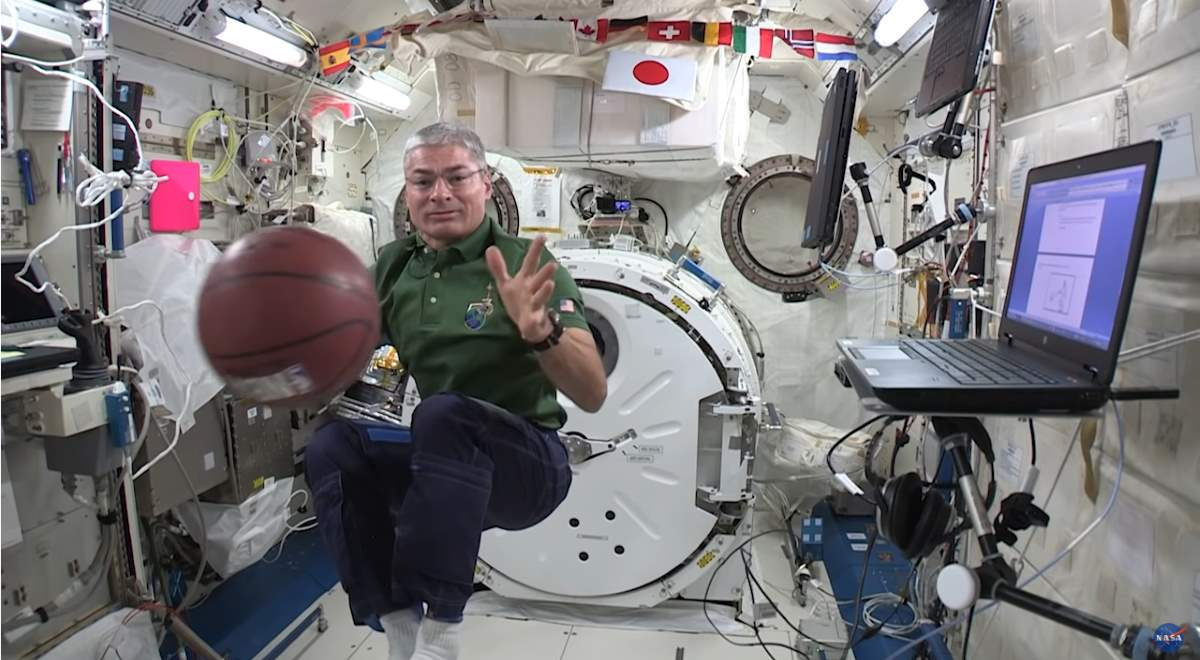A microgravity environment is a perfect place to demonstrate basic physics, i.e. Newton’s laws of motion. In the videos published by the NASA Johnson channel, astronauts aboard the International Space Station (ISS) just do that.

## Newton’s laws of motion

### First Law

In an inertial frame of reference, an object either remains at rest or continues to move at a constant velocity, unless acted upon by a force.

Newton’s first law states that every object will remain at rest or in uniform motion in a straight line unless compelled to change its state by the action of an external force. This is normally taken as the definition of inertia. The key point here is that if there is no net force acting on an object (if all the external forces cancel each other out) then the object will maintain a constant velocity. If that velocity is zero, then the object remains at rest. If an external force is applied, the velocity will change because of the force.

### Second Law

In an inertial frame of reference, the vector sum of the forces F on an object is equal to the mass m of that object multiplied by the acceleration (a) of the object: F = ma. It is assumed here that the mass m is constant.

The second law explains how the velocity of an object changes when it is subjected to an external force. The law defines a force to be equal to a change in momentum (mass times velocity) per change in time. Newton also developed the calculus of mathematics, and the “changes” expressed in the second law are most accurately defined in differential forms. (Calculus can also be used to determine the velocity and location variations experienced by an object subjected to an external force.)

For an object with a constant mass m, the second law states that the force F is the product of an object’s mass and its acceleration a:

F = m * a

For an externally applied force, the change in velocity depends on the mass of the object. A force will cause a change in velocity; and likewise, a change in velocity will generate a force. The equation works both ways.

### Third Law

When one body exerts a force on a second body, the second body simultaneously exerts a force equal in magnitude and opposite in direction on the first body.

The third law states that for every action (force) in nature there is an equal and opposite reaction. In other words, if object A exerts a force on object B, then object B also exerts an equal force on object A. Notice that the forces are exerted on different objects. The third law can be used to explain the generation of lift by a wing and the production of thrust by a jet engine.

This is also how rockets work. A rocket engine produces thrust through action and reaction. The engine produces hot exhaust gases that flow out of the back of the engine. In reaction, a thrusting force is produced in the opposite reaction, and that force moves the rocket and its payload forward.Newton’s laws of motion: NASA astronaut Mark Vande Hei demonstrates Newton’s Third Law of Motion on the International Space Station by exhibiting an equal and opposite force pair using a basketball.

## Sources

Latest posts by M. Özgür Nevres (see all)